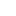# Category: Lesson Plan

## Body Maths: Scatter Graphs

Lesson on scatter graphs inspired by Africa Counts, Claudia Zaslavsky’s excellent book on...

## Writing Functions and Inverse Functions

Find the inverse of a function given by two operations on a number (one multiply by, the other...

## Sequences

Activities where sequences are generated and next, 10th, 100th and nth terms are found. Animations...

## Functions

Lesson where you are given four inputs and two operations (multiply and add or subtract) to create...

## Dice Games

Interactive excel activity that simulates four games of rolling two dice. Originally part of the...

## SS6 Representing 3D Shapes

Super activity from the original Standards Unit Improving Learning in Mathematics but now supplied...

## SS3 Dissecting A Square

Super activity from the original Standards Unit Improving Learning in Mathematics but now supplied...

## Squares and Other Powers

Recognise/use different representations of squares and square roots, cubes and cube roots and...

## Factors and Prime Factors

Recognise/use multiples, factors (divisors), highest common factor, lowest common multiple and...